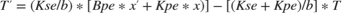# Muscle Reflex Parameter Estimation

This example shows how to estimate parameters of a muscle reflex model.

### Simulink Model of the Muscle Reflex

The Simulink® model for the muscle reflex system, spe_muscle, is shown below.### Muscle Reflex Model Description

For this example a simple knee reflex action of humans is modeled. When the patellar tendon is excited, for example when a doctor strikes it with the nub of a small rubber hammer, the tendon reacts with a small but quick reflex force. This in turn pulls the muscle and we observe that the leg jerks forward slightly at the knee.

For this model we treat the tendon itself as a small torsional spring damper with inertia (J), stiffness (K) and damping (B). When the tendon is excited a signal is sent through the nervous system to the spinal cord reporting a structural change (i.e. tendon length). The nervous system then sends a signal back to the tendon to produce a reflex. There are receptors on the muscle called spindles that have their own dynamics, shown in the model as a transfer function in the feedback path. The spindles are modeled as a spring (Kpe) and damper (Bpe) in parallel, and then with the pair in series with another spring (Kse). The differential equation describing these dynamics is given byFor this model we supply two brief pulses, one stronger than the other, as input. This is similar to what one might experience in a doctor's office.

### Estimation Data

There is a project already associated with this model. You can access it by double-clicking the orange block in the lower left corner of the model. This opens the Parameter Estimator configured with measured experiment data ReflexResponse and parameters J, B, K, Td, beta, alpha, and tau selected for estimation. The measured data in the ReflexResponse experiment is shown in the plot. There is only one data set used for this example.The experiment data can be imported from various sources including MATLAB® variables, MAT files, Excel® files, or comma-separated-value files.

### Estimated Parameters

The estimation parameters are selected by clicking on Select Parameters in the Parameter Estimation tab. We have already loaded the parameters for this model. These parameters are the inertia, J; damping coefficient, B; the return spring constant, K; the neural transmission delay, Td as well as the spindle dynamics parameters beta, alpha, and tau. Since we know from our physical insight that none of these parameters can be negative we set their lower limits to zero. Based on known neural transmission times, we set the lower limit of Td to 10 microseconds.The experiment plot is also used to see how well the measured data matches the current model. Click Plot Model Response to display simulated signal data on the experiment plots. The simulation results show that the model does not match the measured data and that model parameters need to be estimated.### Estimation

With the parameters for estimation specified, we select experiments to use for estimation. Click Select Experiments in the Parameter Estimation tab and select ReflexResponse for estimation.

We are now almost ready to start our estimation but first create another plot to monitor the estimation progress. Click Add Plot and select Parameter Trajectory. This creates a plot that shows how the parameter values change during estimation. Click the arrow to the right of the plot tab and select Tile All to lay out the plots so that the experiment plot and trajectory iteration plot are both visible.

Click the Estimate button in the Parameter Estimation tab to start the estimation. The estimation will keep iterating the parameter values until the estimation converges and terminates. The plot below shows the measured data overlaid with the simulated data. The simulated data comes from the model with the estimated parameters. The results of the estimation appear satisfactory, the simulated curve closely matches the measured results.### Validation

We can also view residuals of the estimation. Residuals are the error between the measured response and simulated response at each time step.

Click the Validation tab and click Select Experiments. Select the ReflexResponse experiment for validation. In the Validation tab, select Plot Residuals and click Validate. The plot below shows that the residuals do not exhibit a correlation pattern. They are one or two orders of magnitude smaller than the measured data and are essentially the noise from the experimental data, so we are again satisfied that parameters in the model were estimated well.The parameters of the model have been tuned to match the experimental results very well and our estimation error is only the original noise in the results. We can conclude that the parameters in the model have been successfully estimated.

Close the model.